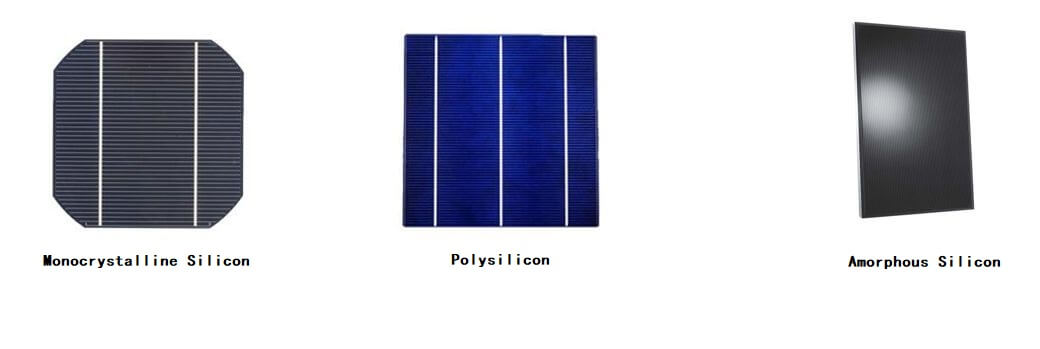# How Much Power Can A 100W Foldable Solar Panel Produce?

Under the premise of good radiation intensity, good light angle, and good solar panel conversion efficiency, a 100w Foldable Solar Panel can generate 0.137kwh electricity in 1 hour, 7.6amp per peak hour.

How do i get these date?

In an ideal state: The generated electricity = solar constant * actual output power / square meter * hour

In actual use: Actual actual output power / square meter = (power consumption * daily power consumption time) / (annual average sunshine time * battery conversion rate)

It can be seen from the above formula that in actual use, the conversion rate of solar panels is determined by many factors:

Irradiation intensity is what we often call the intensity of sunlight. For example, on a sunny day, solar panels receive more sunlight, and at the same conversion rate, the output energy is more. On a cloudy day, the solar panel receives less sunlight and the output energy becomes less.

b. Illumination angle
The angle formed by the Foldable Solar Panel and the sunlight, the better the angle, the best power generation efficiency can be achieved. But the best angle is not the only one. It is determined by the latitude and longitude of your area. The degree of light refraction in different latitudes and longitudes is different, so the best angle is not the same. The picture below shows the latitude and longitude of the continental United States.As can be seen from the above figure, the continental United States lies between latitude 49 north (excluding Alaska) and latitude 26 south (Texas). Having determined the dimensionality, we subtracted the angle of the earth’s tilt 23, 90- (current dimensionality -23). For example, the angle of sunlight in Los Angeles is 90-(34-23)=79, and the solar panel needs to be tilted 90-79=11 to be in vertical contact with the sunlight. In this way, the Foldable Solar Panels will be completely perpendicular to the sun's rays at noon.

c. Foldable Solar Panel materials

At present, the material with the best conversion efficiency is monocrystalline silicon, which can reach a conversion rate of 18%-25%

Monocrystalline silicon solar cell (ITEHIL monocrystalline solar panel)

Monocrystalline silicon solar cells have the highest conversion efficiency. The experimental maximum conversion efficiency is 25%, and the actual conversion efficiency is about 18%. The service life is 15-25 years. Although the cost is high, it still occupies large-scale applications and industrial production leading position.

Polycrystalline silicon solar cell

The conversion efficiency of polycrystalline silicon solar cells is slightly lower. The maximum conversion efficiency in the experiment is 20%, and the actual conversion efficiency is about 15%. The service life is lower than that of monocrystalline silicon, and the cost is low. At present, many photovoltaic manufacturers have mass-produced polysilicon.

Amorphous silicon solar cell

Amorphous silicon solar cells have low cost and good low-light effect, but the conversion efficiency is less than 10%, the service life is short, and the material with poor stability can easily cause the photoelectric efficiency decline effect, which affects its actual use.

How to distinguish monocrystalline silicon, polycrystalline silicon, and amorphous silicon cells?

From the appearance point of view, the four corners of the monocrystalline silicon battery are arc-shaped and the surface has no patterns; while the four corners of the polycrystalline silicon battery are square corners with a pattern similar to ice flowers on the surface; the amorphous silicon battery is what we usually say Unlike the crystalline silicon cell, which can see the grid lines, the surface is as clear and smooth as a mirror.d. Surface temperature of 100w Foldable Solar Panel

With a constant temperature of 25 degrees Celsius as the standard, the power will drop by 0.32% for every temperature rise of 1 degree Celsius

PS: For a fixed solar panel, winter is when its power is at its maximum.

Under ideal conditions, ITEHIL 100w 18V Foldable solar panel can produce 0.137kwh (that is, 7.6amp) in 1 hour

The generated electricity = solar constant * actual output power / square meter * hour

• Solar Constant = 1.367kw
• Actual output power = Foldable Solar Panel Power = 100w = 0.1kw
• Hour = 1

The generated electricity = 1.367kw*0.1kw*1h=0.137kwh

Electricity generated in 1 hour = The generated electricity/Foldable solar panel voltage = 137wh/18v = 7.6amp

(The unit of kwh needs to be converted into watt-hour, which is 0.137kwh*1000=137wh)

In actual use, only about 70% of the electricity generated by the foldable solar panel can be used. In the conversion process of various electrical appliances, more or less energy will be lost due to resistance, so in actual use you can only Measured 0.137kwh*70%=0.096kwh (7.6amp*70%=5.36amp).